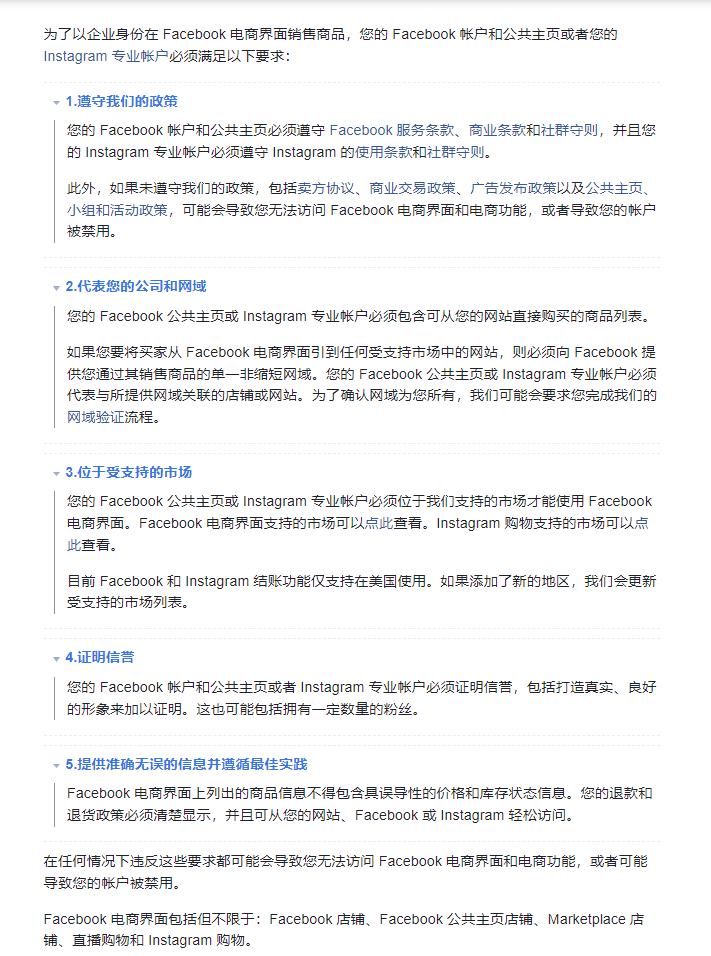【Instagram Shop受支持的市场】参考链接：https://help.instagram.com/321000045119159/?helpref=uf_share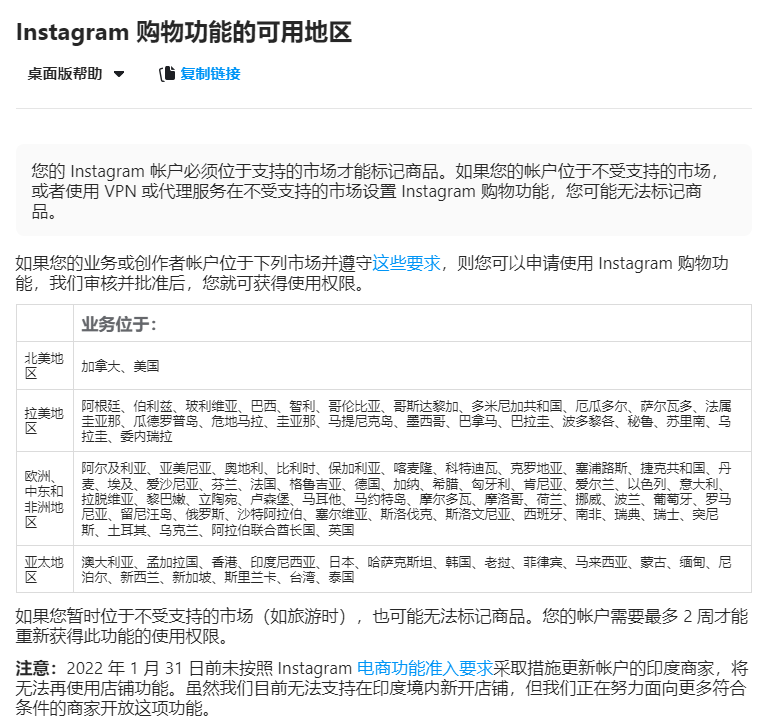• 美国
• 加拿大
• 美国
• 加拿大
• 美国

• 阿根廷
• 玻利维亚
• 巴西
• 智利
• 哥伦比亚
• 哥斯达黎加
• 多米尼加共和国
• 厄瓜多尔
• 萨尔瓦多
• 危地马拉
• 尼加拉瓜
• 墨西哥
• 巴拿马
• 巴拉圭
• 秘鲁
• 波多黎各
• 乌拉圭
• 委内瑞拉
• 安圭拉
• 安提瓜和巴布达
• 阿根廷
• 阿鲁巴
• 巴哈马
• 巴巴多斯
• 伯利兹
• 百慕大
• 玻利维亚
• 巴西
• 开曼群岛
• 智利
• 哥伦比亚
• 哥斯达黎加
• 古巴
• 多米尼克
• 多米尼加共和国
• 厄瓜多尔
• 萨尔瓦多
• 福克兰群岛
• 格林纳达
• 危地马拉
• 圭亚那
• 海地
• 洪都拉斯
• 牙买加
• 墨西哥
• 蒙塞拉特岛
• 尼加拉瓜
• 巴拿马
• 巴拉圭
• 秘鲁
• 波多黎各
• 圣基茨和尼维斯
• 圣卢西亚
• 圣文森特和格林纳丁斯
• 苏里南
• 特立尼达和多巴哥
• 特克斯和凯科斯群岛
• 乌拉圭
• 委内瑞拉
• 美属维尔京群岛

• 阿尔及利亚
• 亚美尼亚
• 奥地利
• 比利时
• 保加利亚
• 喀麦隆
• 科特迪瓦
• 克罗地亚
• 塞浦路斯
• 捷克
• 丹麦
• 埃及
• 爱沙尼亚
• 芬兰
• 法国
• 格鲁吉亚
• 德国
• 加纳
• 希腊
• 根西岛
• 匈牙利
• 伊拉克
• 爱尔兰
• 马恩岛
• 以色列
• 意大利
• 泽西岛
• 哈萨克斯坦
• 肯尼亚
• 拉脱维亚
• 黎巴嫩
• 立陶宛
• 马耳他
• 摩尔多瓦
• 摩洛哥
• 荷兰
• 挪威
• 巴基斯坦
• 波兰
• 葡萄牙
• 罗马尼亚
• 俄罗斯
• 沙特阿拉伯
• 塞尔维亚
• 斯洛伐克
• 斯洛文尼亚
• 南非
• 西班牙
• 瑞典
• 瑞士
• 突尼斯
• 土耳其
• 乌克兰
• 阿拉伯联合酋长国
• 英国
• 阿富汗
• 阿尔巴尼亚
• 阿尔及利亚
• 安道尔
• 安哥拉
• 亚美尼亚
• 奥地利
• 阿塞拜疆
• 巴林
• 白俄罗斯
• 比利时
• 贝宁
• 博奈尔
• 圣尤斯特歇斯和沙巴
• 波斯尼亚和黑塞哥维那
• 博茨瓦纳
• 保加利亚
• 布基纳法索
• 布隆迪
• 佛得角
• 喀麦隆
• 中非共和国
• 乍得
• 科摩罗
• 刚果
• 刚果民主共和国
• 库克群岛
• 科特迪瓦
• 克罗地亚
• 库拉索
• 塞浦路斯
• 捷克
• 丹麦
• 吉布提
• 埃及
• 赤道几内亚
• 厄立特里亚
• 爱沙尼亚
• 斯威士兰
• 埃塞俄比亚
• 法罗群岛
• 芬兰
• 法国
• 法属圭亚那
• 法属玻利尼西亚
• 加蓬
• 冈比亚
• 格鲁吉亚
• 德国
• 加纳
• 直布罗陀
• 希腊
• 格陵兰
• 瓜德罗普岛
• 根西岛
• 几内亚
• 几内亚比绍
• 匈牙利
• 冰岛
• 伊朗
• 伊拉克
• 爱尔兰
• 马恩岛
• 以色列
• 意大利
• 泽西岛
• 约旦
• 哈萨克斯坦
• 肯尼亚
• 科威特
• 吉尔吉斯斯坦
• 拉脱维亚
• 黎巴嫩
• 莱索托
• 利比里亚
• 利比亚
• 列支敦士登
• 立陶宛
• 卢森堡
• 马其顿
• 马达加斯加
• 马拉维
• 马里
• 马耳他
• 马提尼克岛
• 毛里塔尼亚
• 毛里求斯
• 马约特
• 摩尔多瓦
• 摩纳哥
• 黑山共和国
• 摩洛哥
• 莫桑比克
• 纳米比亚
• 荷兰
• 新喀里多尼亚
• 尼日尔
• 挪威
• 阿曼
• 巴勒斯坦
• 波兰
• 葡萄牙
• 卡塔尔
• 留尼汪岛
• 罗马尼亚
• 俄罗斯
• 卢旺达
• 圣巴泰勒米
• 圣赫勒拿、阿森松和特里斯坦 - 达库尼亚群岛
• 圣马丁
• 圣皮埃尔和密克隆
• 圣马力诺
• 圣多美和普林西比
• 沙特阿拉伯
• 塞内加尔
• 塞尔维亚
• 塞舌尔
• 塞拉利昂
• 圣马丁（荷属）
• 斯洛伐克
• 斯洛文尼亚
• 索马里
• 南非
• 南苏丹
• 西班牙
• 苏丹
• 斯瓦尔巴群岛和扬马延岛
• 瑞典
• 瑞士
• 阿拉伯叙利亚共和国
• 塔吉克斯坦
• 坦桑尼亚
• 多哥
• 突尼斯
• 土耳其
• 土库曼斯坦
• 乌干达
• 乌克兰
• 阿拉伯联合酋长国
• 英国
• 乌兹别克斯坦
• 瓦利斯和富图纳群岛
• 西撒哈拉
• 也门
• 赞比亚
• 津巴布韦

• 澳大利亚
• 孟加拉国
• 柬埔寨
• 香港
• 印度尼西亚
• 日本
• 韩国
• 老挝
• 马来西亚
• 蒙古
• 缅甸
• 尼泊尔
• 新西兰
• 巴基斯坦
• 菲律宾
• 新加坡
• 斯里兰卡
• 台湾
• 泰国
• 美属萨摩亚
• 澳大利亚
• 孟加拉国
• 不丹
• 文莱达鲁萨兰国
• 柬埔寨
• 圣诞岛
• 斐济
• 关岛
• 香港
• 印度尼西亚
• 日本
• 基里巴斯
• 韩国
• 老挝
• 澳门
• 马来西亚
• 马尔代夫
• 马绍尔群岛
• 密克罗尼西亚
• 蒙古
• 缅甸
• 瑙鲁
• 尼泊尔
• 新西兰
• 纽埃
• 诺福克岛
• 北马里亚纳群岛
• 巴基斯坦
• 帕劳
• 巴布亚新几内亚
• 菲律宾
• 萨摩亚
• 新加坡
• 所罗门群岛
• 斯里兰卡
• 台湾
• 泰国
• 东帝汶
• 托克劳
• 汤加
• 图瓦卢
• 瓦努阿图

*截至 2022 年 4 月，根据美国、英国和欧盟实施的国际法规，Meta 已限制在克里米亚、塞瓦斯托波尔、卢甘斯克人民共和国（“LNR”）和顿涅茨克人民共和国（“DNR”）地区的商业活动。位于这些地区的商家将无法访问其电商管理工具帐户，但是他们的帐户和店铺仍可使用。

(1)：我们的账号是在国内使用大陆手机号和英国VPN注册的，但还是无法开通Shop功能。

(2)：我们的账号是在国内使用谷歌邮箱和美国VPN注册，但还是无法开通Shop功能。

(3)：我们的账号是在国内某宝购买的英国手机号和英国VPN注册的，但还是无法开通Shop功能。

(4)：我们的账号是在国内使用日本手机号和日本VPN注册，但还是无法开通Shop功能。

3、常见问题

3.1、如何注册美国账号？（其他区域同理）

① 使用的邮箱必须要使用美国的邮箱，如果使用的邮箱是国内的邮箱比如QQ、163等，那么大概率会被认定为国内账号。

② 使用的手机号必须要使用美国的手机号，如使用国内手机，比如+86开通，那么也会被认定为国内账号。

③ 填写的个人信息资料必须要填写美国所在信息，如果有填写国内的大概率就会被认定为国内账号。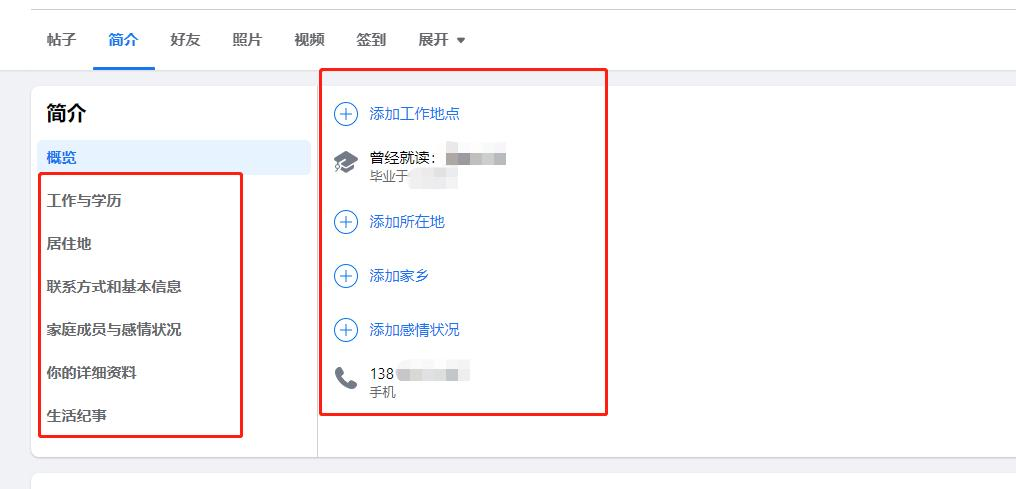④ 添加的好友需要大多数为美国的，如果有很多是国内用户，那么大概率也会被认定为国内账号。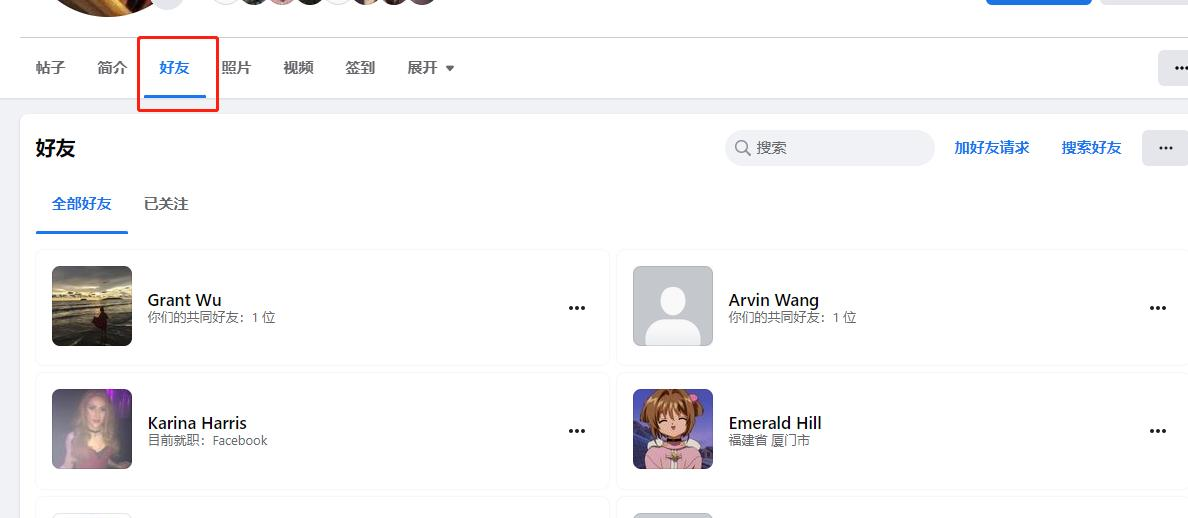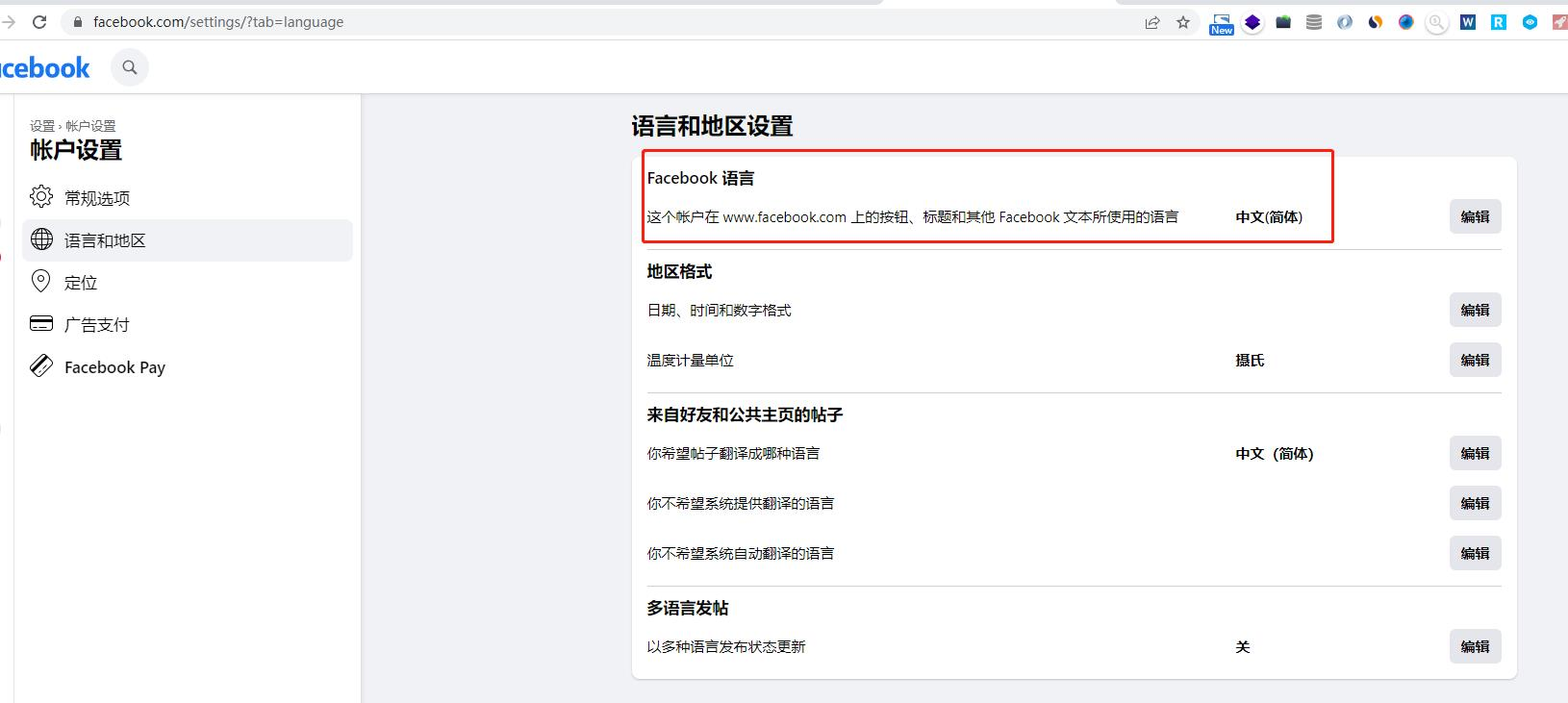Instagram专业账号在开通Shop功能后，可以尝试在中国大陆登录，切记不要频繁换IP。

3.3、成功开通Instagram Shop后，后续在中国大陆登录Instagram专业账号，然后Shop功能被撤销了，这是什么原因呀？

A：这是因为系统监测到你在中国大陆使用Instagram专业账号，而中国大陆不支持Shop功能。还有如果你暂时位于不受支持的市场（如旅游时），也可能无法标记商品。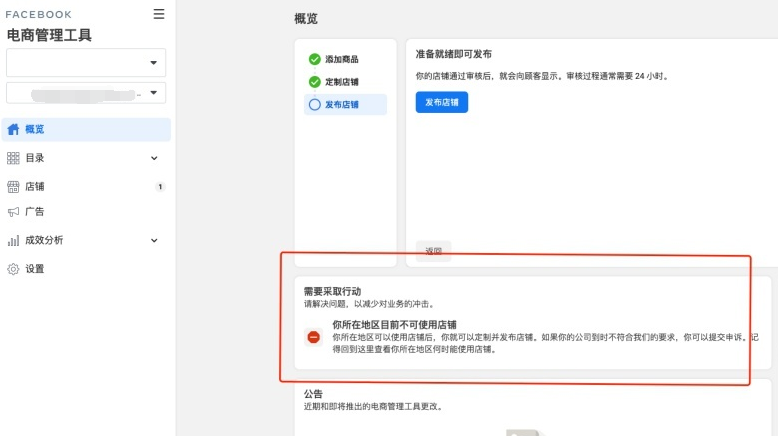PS：如果电商管理工具出现上图红框的内容，原因基本就是账号的注册归属地不位于受支持的市场。

3.4、账号是请朋友帮忙在英国当地使用英国手机号注册的，运营团队都在中国大陆，平时需要运营维护账号，这种情况怎么办呢？

A：这种方式一般会归属于美国的，但最稳妥的做法还是建议使用手机号注册。

• 最后更新于 2022-12-02

## 来自客户的评价##### 张小姐##### 婴儿之家